﻿ Free Single Digit Addition Worksheets

Free Single Digit Addition Fluency Worksheets

Single Digit Addition Fluency Worksheets, Doubles Worksheets, Doubles Plus One, .....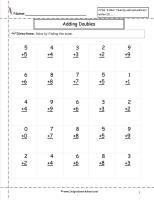Doubles Addition Facts Worksheet

Common Core State Standards: 2.OA.2 Add and Subtract within 20.
25 doubles problems.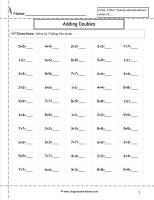Doubles Addition Facts Worksheet

Common Core State Standards: 2.OA.2 Add and Subtract within 20.
50 doubles problems.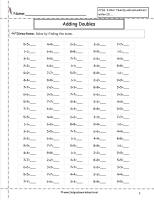Doubles Addition Facts Worksheet

Common Core State Standards: 2.OA.2 Add and Subtract within 20.
100 doubles problems.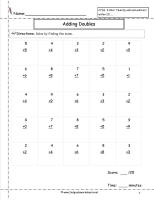Doubles Addition Facts Worksheet Timed

Common Core State Standards: 2.OA.2 Add and Subtract within 20.
25 doubles problems timed.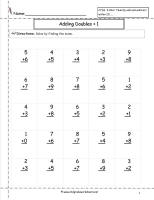Doubles Plus One Addition Facts Worksheet

Common Core State Standards: 2.OA.2 Add and Subtract within 20.
25 doubles plus one problems.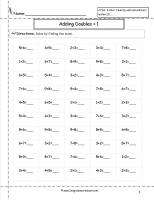Doubles Plus One Addition Facts Worksheet

Common Core State Standards: 2.OA.2 Add and Subtract within 20.
50 doubles plus one problems.Doubles Plus One Addition Facts Worksheet

Common Core State Standards: 2.OA.2 Add and Subtract within 20.
100 doubles plus one problems.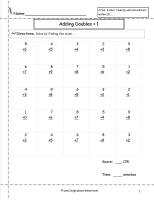Doubles Plus One Addition Facts Worksheet Timed

Common Core State Standards: 2.OA.2 Add and Subtract within 20.
25 doubles plus one problems timed.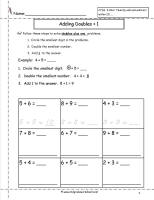Doubles Plus One Intro Addition Facts Worksheet

Common Core State Standards: 2.OA.2 Add and Subtract within 20.
Introduction to doubles plus one problems.Adding Zero Addition Facts Worksheet

Common Core State Standards: 2.OA.2 Add and Subtract within 20.Adding Zero and One Addition Facts Worksheet

Common Core State Standards: 2.OA.2 Add and Subtract within 20.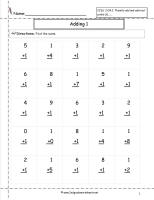Adding One Addition Facts Worksheet

Common Core State Standards: 2.OA.2 Add and Subtract within 20.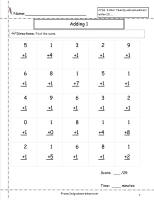Adding One Addition Facts Worksheet Timed

Common Core State Standards: 2.OA.2 Add and Subtract within 20.Adding One and Two Addition Facts Worksheet

Common Core State Standards: 2.OA.2 Add and Subtract within 20.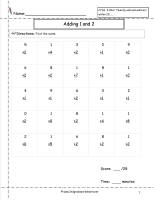Adding one and Two Addition Facts Worksheet Timed

Common Core State Standards: 2.OA.2 Add and Subtract within 20.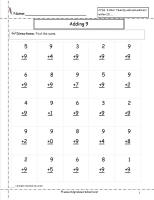Adding Nine Addition Facts Worksheet

Common Core State Standards: 2.OA.2 Add and Subtract within 20.Adding Nine Addition Facts Worksheet

Common Core State Standards: 2.OA.2 Add and Subtract within 20.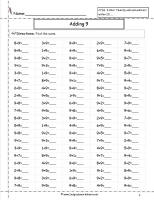Adding Nine Addition Facts Worksheet

Common Core State Standards: 2.OA.2 Add and Subtract within 20.Adding Nine Addition Facts Worksheet Timed

Common Core State Standards: 2.OA.2 Add and Subtract within 20.Adding Ten Addition Facts Worksheet

Common Core State Standards: 2.OA.2 Add and Subtract within 20.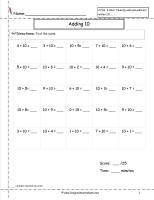Adding Ten Addition Facts Worksheet Timed

Common Core State Standards: 2.OA.2 Add and Subtract within 20.Adding Ten Addition Facts Worksheet

Common Core State Standards: 2.OA.2 Add and Subtract within 20.
Adding ten worksheet with word problems.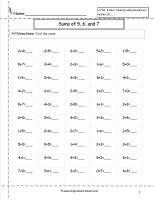Sums of Five, Six, and Seven Addition Facts Worksheet

Common Core State Standards: 2.OA.2 Add and Subtract within 20.
50 problems sums of five, six and seven.Sums of Five, Six, and Seven Addition Facts Worksheet

Common Core State Standards: 2.OA.2 Add and Subtract within 20.
100 problems sums of five, six and seven.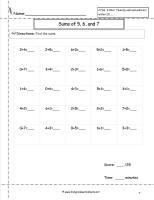Sums of Five, Six, and Seven Addition Facts Worksheet

Common Core State Standards: 2.OA.2 Add and Subtract within 20.
25 problems sums of five, six and seven timed.Sums of Eight and Nine Addition Facts Worksheet

Common Core State Standards: 2.OA.2 Add and Subtract within 20.
25 problems sums of eight and nine.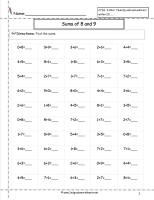Sums of Eight and Nine Addition Facts Worksheet

Common Core State Standards: 2.OA.2 Add and Subtract within 20.
50 problems sums of eight and nine.Sums of Eight and Nine Addition Facts Worksheet

Common Core State Standards: 2.OA.2 Add and Subtract within 20.
100 problems sums of eight and nine.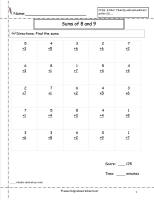Sums of Eight and Nine Addition Facts Worksheet

Common Core State Standards: 2.OA.2 Add and Subtract within 20.
25 problems sums of eight and nine timed.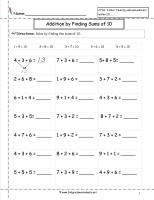Sums of Ten Addition Facts Worksheet

Common Core State Standards: 2.OA.2 Add and Subtract within 20.
Finding the sums of ten worksheet.  This worksheet includes sums of ten cues at the top.  20 problems.Addition Facts to 10

Common Core State Standards: 2.OA.2 Add and Subtract within 20.
Addition facts to sum of 10.  20 pages.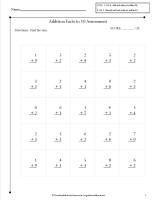Addition Facts to 10 Assessment

Common Core State Standards: 2.OA.2 Add and Subtract within 20.
Addition facts to sum of 10.  10 pages of assessments.Addition Facts to 20

Common Core State Standards: 2.OA.2 Add and Subtract within 20.
Addition facts to sum of 20.  20 pages.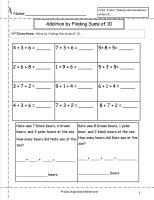Addition Facts to 20 Assessments

Common Core State Standards: 2.OA.2 Add and Subtract within 20.
Addition facts to sum of 20.  10 pages of assessments.Sums of Ten Addition Facts Worksheet

Common Core State Standards: 2.OA.2 Add and Subtract within 20.
Finding the sums of ten worksheet with word problems..Sums of Ten Addition Facts Worksheet

Common Core State Standards: 2.OA.2 Add and Subtract within 20.
Finding the sums of ten worksheet with word problems..Sums of Ten Addition Facts Worksheet

Common Core State Standards: 2.OA.2 Add and Subtract within 20.
Finding the sums of ten worksheet with word problems..Sums of Ten Addition Facts Worksheet

Common Core State Standards: 2.OA.2 Add and Subtract within 20.
Finding the sums of ten worksheet with word problems..Sums of Ten Addition Facts Worksheet

Common Core State Standards: 2.OA.2 Add and Subtract within 20.
Finding the sums of ten worksheet with word problems..